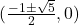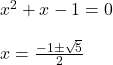## Find the points of intersection of the graphs involving the following pair of functions. f(x)=2x^2 + 3x – 3 and g(x) = -x^2

Question

Find the points of intersection of the graphs involving the following pair of functions.

f(x)=2x^2 + 3x – 3 and g(x) = -x^2

in progress 0
1 month 2021-08-04T08:40:36+00:00 1 Answers 1 views 0

The point of intersection isStep-by-step explanation:

f(x) = 2x^2 + 3x – 3 and g(x) = – x^2

By equating them

2x^2 + 3x – 3 = -x^2

3x^2 + 3 x – 3 =  0

x^2 + x – 1 = 0